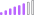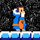cancel
Showing results for
Did you mean:Post Patron

## SUM of Count of Distinct Values

Hello,

I have a column [Name] full of names (text).

I'm my visualization I am applying the 'Count Distinct' summarization. I would like to create a measure that will simply SUM the total of distinct names, but I can't figure it out at all.

Any help would be greatly appreciated.

1 ACCEPTED SOLUTIONSuper User

Try the following formula:

``````User Distinct =
VAR t =
SUMMARIZE (
'Table',
Table[Date],
"UserDistinct", DISTINCTCOUNT ( Table[Name] )
)
RETURN
SUMX ( t, [UserDistinct] )``````
6 REPLIES 6Hi, I'd like help in a similar DAX formula.

To one extent I'm calculating: the distinctive count where the sale is greater than 0 of a string field.

I would like to get the total or sum of that count, since the result is not adding the values of each row to me.

I look forward to your help, thank you very muchSuper User

Try the following formula:

``````User Distinct =
VAR t =
SUMMARIZE (
'Table',
Table[Date],
"UserDistinct", DISTINCTCOUNT ( Table[Name] )
)
RETURN
SUMX ( t, [UserDistinct] )``````That's great! your formula has worked for me. I had the same problem.Post Patron

That seems to have done it! Thank you so much.Super User

Hi. What do you mean with SUM the total of distinct names?

You you just want the number of the "Count Distinct" summarization you can write a dax measure like

``Measure = DISTINCTCOUNT ( Table[Column] )``

Hope that helps,

If this post helps, then please consider Accept it as the solution to help the other members find it more quickly.

Happy to help!Post Patron

Thanks for your response. I'll try to clarify.

If I look at a date, say 1/21/2021, using Count Distinct I am returned 90 unique names. If I go a day earlier, I see 85 unique names.

If I select two days, I still only see 90 unique names, where I would like to see the SUM of both of those days'-worth unique names.